Question

# Consider a continuous random variable X with the density function (exponential) ?(?)={?^−? ?? ?≥0 , 0...

Consider a continuous random variable X with the density function (exponential) ?(?)={?^−? ?? ?≥0 , 0 ??ℎ??????}
a) Find and sketch the CDF for X
b) Find the mean and variance of X (I want to see your calculation)
c) Find ?(1≤?≤2)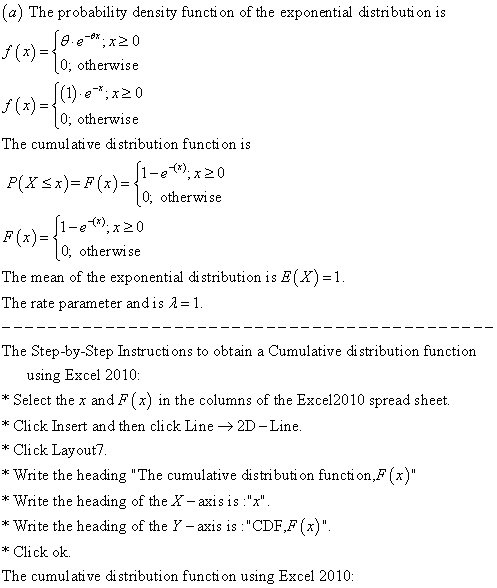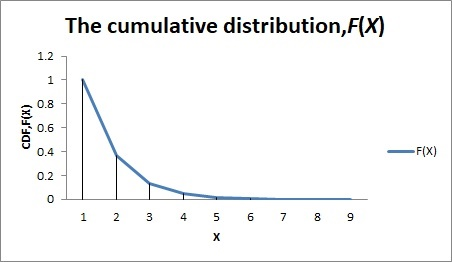Interpretation:

The measures of the central tendency of the exponential distribution are mean and variance. The mean of the exponential distribution is E(X)=1, the parameter of the exponential distribution is . The variance of the exponential distribution is Var(X)=1.

-------------------------------------------------------------------------------------------------------------------------------------------------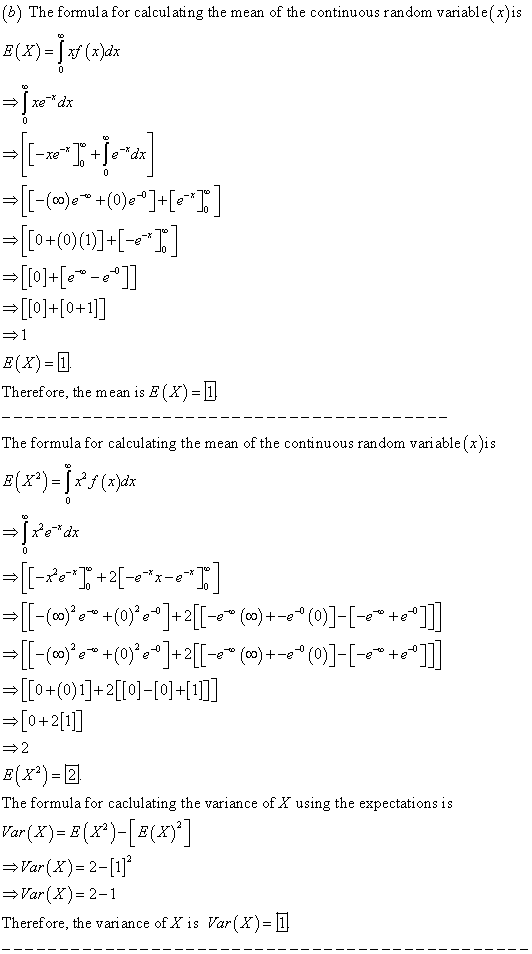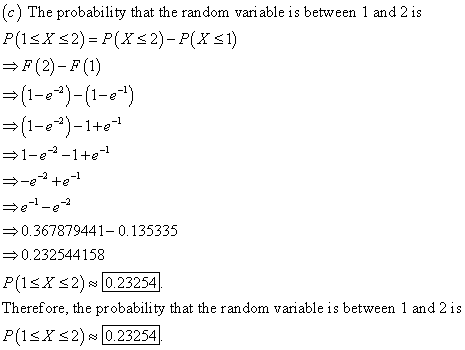#### Earn Coins

Coins can be redeemed for fabulous gifts.

Similar Homework Help Questions
• ### is a continuous random variable with the probability density function (x) = { 4x 0 <=...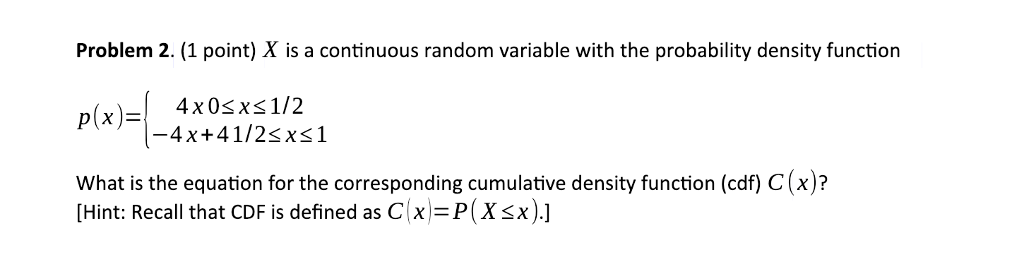is a continuous random variable with the probability density function (x) = { 4x 0 <= x <= 1/2 { -4x + 4 1/2 <= x <= 1 What is the equation for the corresponding cumulative density function (cdf) C(x)? [Hint: Recall that CDF is defined as C(x) = P(X<=x).] We were unable to transcribe this imageWe were unable to transcribe this imageProblem 2. (1 point) X is a continuous random variable with the probability density function -4x+41/2sxs1 What is...

• ### (1) Suppose that X is a continuous random variable with probability density function 0<x< 1 f()...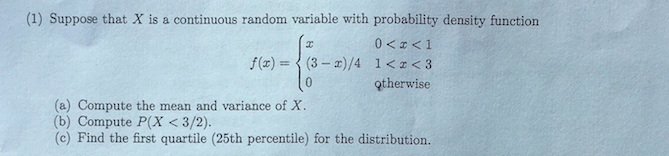(1) Suppose that X is a continuous random variable with probability density function 0<x< 1 f() = (3-X)/4 i<< <3 10 otherwise (a) Compute the mean and variance of X. (b) Compute P(X <3/2). (c) Find the first quartile (25th percentile) for the distribution.

• ### For a continuous random variable X with the following probability density function (PDF): fX(x) = (...

For a continuous random variable X with the following probability density function (PDF): fX(x) = ( 0.25 if 0 ≤ x ≤ 4, 0 otherwise. (a) Sketch-out the function and confirm it’s a valid PDF. (5 points) (b) Find the CDF of X and sketch it out. (5 points) (c) Find P [ 0.5 < X ≤ 1.5 ]. (5 points)

• ### Consider a continuous random variable X with the following probability density function: Problem 2 (15 minutes)...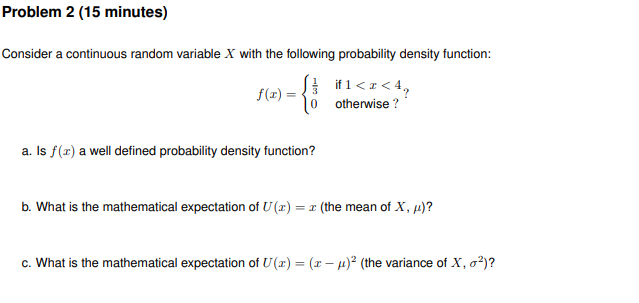Consider a continuous random variable X with the following probability density function: Problem 2 (15 minutes) Consider a continuous random variable X with the following probability density function: f(x) = {& Otherwise ?' 10 otherwise? a. Is /(x) a well defined probability density function? b. What is the mathematical expectation of U (2) = x (the mean of X, )? c. What is the mathematical expectation of U(z) = (1 - 2 (the variance of X, oº)?

• ### A continuous random variable X has the probability density function f(x) = e^(-x), x>0 a) Compute...

A continuous random variable X has the probability density function f(x) = e^(-x), x>0 a) Compute the mean and variance of this random variable. b) Derive the probability density function of the random variable Y = X^3. c) Compute the mean and variance of the random variable Y in part b)

• ### 9.) Suppose that X is a continuous random variable with density C(1- if r [0,1 0...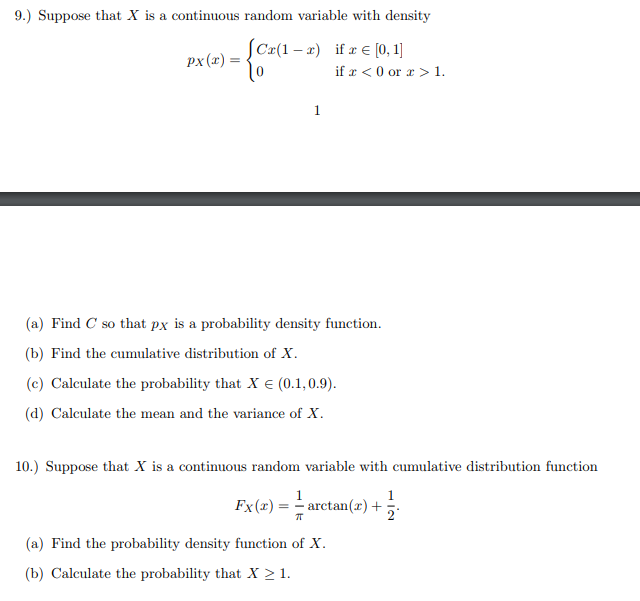9.) Suppose that X is a continuous random variable with density C(1- if r [0,1 0 ¡f x < 0 or x > 1. (a) Find C so that px is a probability density function (b) Find the cumulative distribution of X (c) Calculate the probability that X є (0.1,0.9). (d) Calculate the mean and the variance of X 10.) Suppose that X is a continuous random variable with cumulative distribution function Fx()- arctan()+ (a) Find the probability density function...

• ### 3. Let X be a continuous random variable with probability density function ax2 + bx f(0)...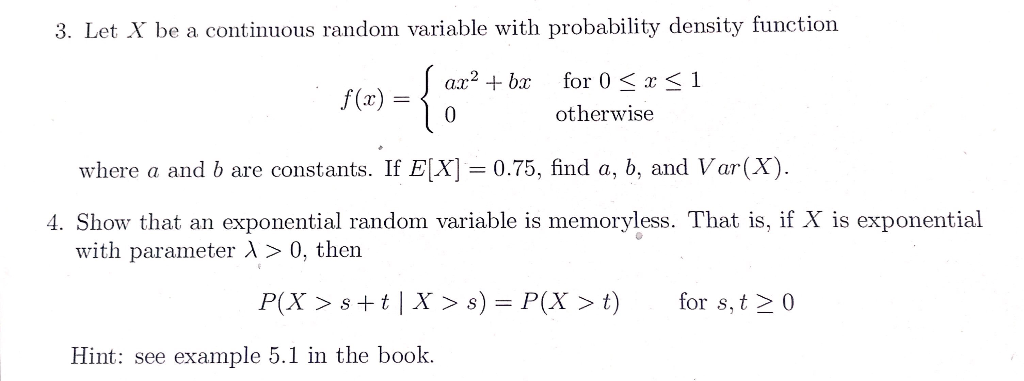3. Let X be a continuous random variable with probability density function ax2 + bx f(0) = -{ { for 0 < x <1 otherwise 0 where a and b are constants. If E(X) = 0.75, find a, b, and Var(X). 4. Show that an exponential random variable is memoryless. That is, if X is exponential with parameter > 0, then P(X > s+t | X > s) = P(X > t) for s,t> 0 Hint: see example 5.1 in...

• ### Suppose that X is a continuous random variable with density pX(x) = ( Cx(1 − x)...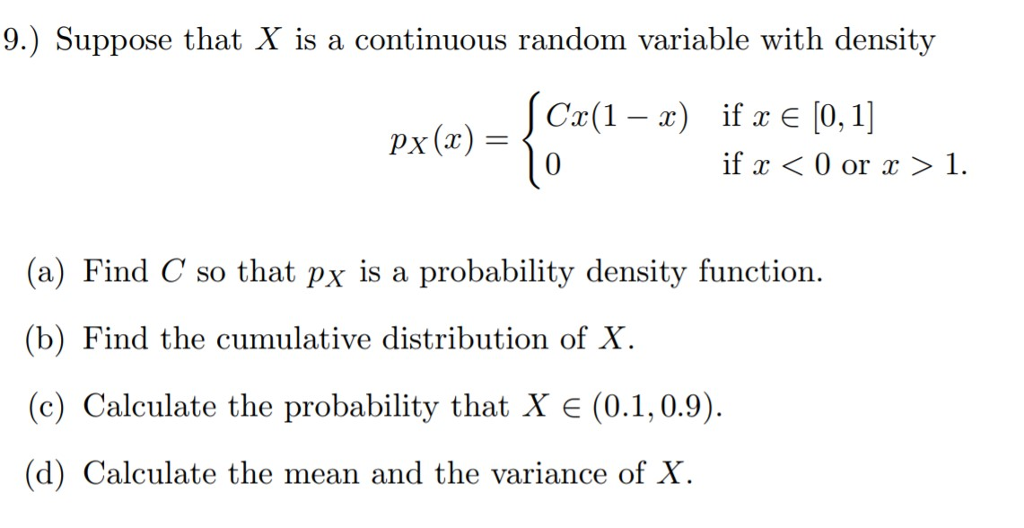Suppose that X is a continuous random variable with density pX(x) = ( Cx(1 − x) if x ∈ [0, 1] 0 if x < 0 or x > 1. (a) Find C so that pX is a probability density function. (b) Find the cumulative distribution of X. (c) Calculate the probability that X ∈ (0.1, 0.9). (d) Calculate the mean and the variance of X. 9.) Suppose that X is a continuous random variable with density C(1x) if E...

• ### 5. Find the moment generating function of the continuous random variable X whose a. probability density...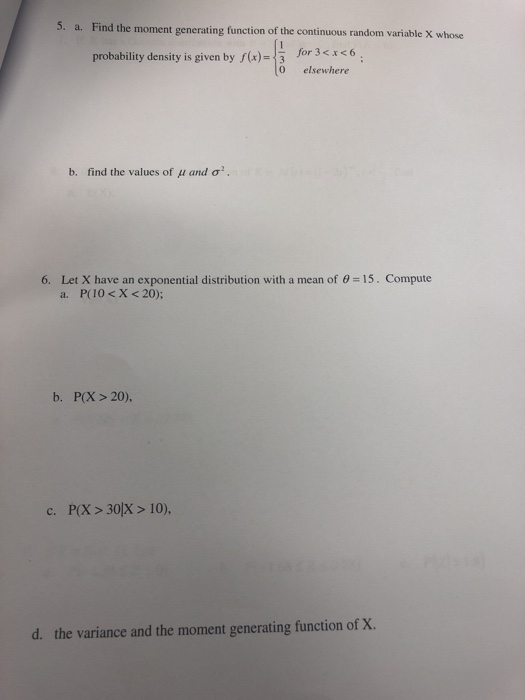5. Find the moment generating function of the continuous random variable X whose a. probability density is given by )-3 or 36 0 elsewhere find the values of μ and σ2. b, Let X have an exponential distribution with a mean of θ = 15 . Compute a. 6. P(10 < X <20); b. P(X>20), c. P(X>30X > 10), the variance and the moment generating function of x. d.

• ### The cumulative distribution function for a continuous random variable X is given by 0, S 0...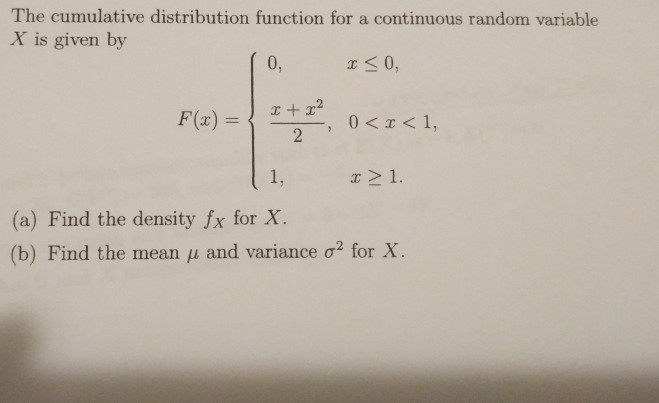The cumulative distribution function for a continuous random variable X is given by 0, S 0 F(x) = 1, r 21. (a) Find the density fx for X. (b) Find the mean ? and variance ?2 for X.